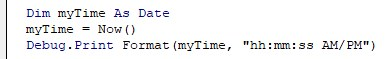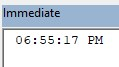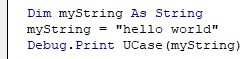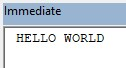# VBA FormatArticle byExcelMojo Team## What Is Excel VBA Format Function?

The Excel VBA Format() function is used to format a numeric or string expression in a specific format. It returns a string representing the formatted value specified in the input expression.

To fully utilize the VBA format conditions, one should be aware of its many uses in formatting data to a more understandable, readable format for clients or other purposes. Formatting can range from adding numbers with commas to changing dates to other forms. The Format() function can format numbers, dates, times, and currency values. For example, you can use the following code to format a date value:

Here, today’s date will be printed in the popup box in the format specified.

###### Key Takeaways
• The VBA Format function converts a value (such as a number or date) into a string with a specific format. You can use format codes to specify how the output string should appear (e.g., number of decimal places, date format, etc.).
• The function returns a string representing the formatted value and does not modify the original input expression. It can format different data types, including numbers, dates, times, and text.
• The VBA Format function is powerful but complex, requiring some knowledge of format codes to use effectively. It is important to ensure the input value is valid, especially when formatting dates and times.

### Syntax Of VBA Format

The syntax of the VBA Format function is as follows.

Here’s a brief explanation of the arguments:

• expression (required): The expression that you want to format.
• format (optional): The format code you want to apply to the expression. If you omit this argument, the function uses the default format for the expression’s data type.
• firstdayofweek (optional): A number specifying the week’s first day. If you omit this argument, the function uses the system’s default value.
• firstweekofyear (optional): A number specifying the year’s first week. If you omit this argument, the function uses the system’s default value.

### How To Use Excel VBA Format?

To access the VBA Format text command, write the necessary code to implement it and keep it ready.

Step 1: Go to the Developer” tab in the toolbar and click the “Visual Basic” option. Now, the VBA Editor opens to add functions and subprocedures. Next, click the “Insert” button followed by the “Module” to create a new module or blank page.

Step 2: Consider an example where you want to display today’s date in the “MM/DD/YYYY” format. It can be done by modifying the VBA Format conditions from “DD/MM/YYYY” (Excel’s default representation) to “MM/DD/YYYY.”

Step 3: Create a function called FormatDate() to change the date format in excel .

Step 4: Define a variable called “myDate” with the datatype Date.

Step 5: Initialize a value for myDate using the DateSerial(), which accepts dates in the “DD/MM/YYYY” format.

Step 6: Print it in the immediate terminal using the Debug.Print function. Apply the VBA Format function and print it again to compare and contrast.

Step 7: Run the code by pressing the F5 or Run button. It will print as seen below.

The first print will print the date in the default format of Excel, that is, “DD/MM/YYYY” and the second print will the date in the formatted way as “MM/DD/YYYY” as shown.

Code:

Sub FormatDate()
Dim myDate As Date
myDate = DateSerial(2023, 4, 1)
Debug.Print myDate
Debug.Print Format(myDate, “mm/dd/yyyy”)
End Sub

Other Formats that can be changed using the Format Function

#### Currency Format

Consider the table:

Here, you must add the currency and separate the number using the VBA format number to make it more readable.

Step 1: In the VBA Editor, declare a function FormatFinancialData() that formats the above numbers.

Step 2: In the function, initialize variables rngRevenue, rngExpenses, and rngProfit as the datatype Range and ws as the datatype Worksheet (to define the sheet number it should work in).

Step 3: Define values for all the variables mentioned above. Their revenue, expenses, and profit ranges are from A2-A10, B2-B10, and C2-C10, respectively.

The worksheet where this function will be applied is mentioned as Sheet1.

Step 4: Initialize a FOR loop throughout the range of the table to format it into currency by adding “\$” before the number for the currency symbol.

• Here, rngRevenue.Rows.Count represents the count of rows in the range, rngRevenue.
• Using “i,” each row’s number is formatted as “S#,##0.00” representing the currency in dollars in decimal values.
• The comma is added to separate the numbers based on the American numerical system.

Step 5: To execute the code, click the green arrow button on the VBA toolbar.

Code:

Sub FormatFinancialData()
Dim rngRevenue As Range
Dim rngExpenses As Range
Dim rngProfit As Range
Dim ws As Worksheet
Set ws = ThisWorkbook.Sheets(“Sheet1”)
Set rngRevenue = Range(“A2:A10”)
Set rngExpenses = Range(“B2:B10”)
Set rngProfit = Range(“C2:C10”)
For i = 1 To rngRevenue.Rows.Count
rngRevenue.Cells(i).Value = Format(rngRevenue.Cells(i).Value, “\$#,##0.00”)
rngExpenses.Cells(i).Value = Format(rngExpenses.Cells(i).Value, “\$#,##0.00”)
rngProfit.Cells(i).Value = Format(rngProfit.Cells(i).Value, “\$#,##0.00”)
Next i
End Sub

Step 6: The output is shown below.

Please note that Excel will automatically convert to the national currency of any country you currently use it in. So, for instance, if your system is configured with Japan settings, you will get the Yen symbol (¥).

#### Fixed Format

Consider a table:

We must format it to display only up to one decimal point. For this, we will use the VBA format number.

Step 1: To begin with formatting, create a procedure FormatFixedData() that formats the numbers as mentioned above.

Step 2: Inside the procedure, initialize variables rngData as Range datatype and ws as Worksheet.

Step 3: Initialize values for both the variables mentioned above. Set rngData to the size or range of the table. In this case, it is from A2:A10. Next, set the worksheet to the current one you’re working on. In this case, it is “Sheet2.”

Step 4: Initialize a FOR loop which loops through the entire table iteratively and formats the numbers up to one decimal. The VBA Format function formats all cell values up to one decimal place.

Step 5: Press the green triangle on the VBA toolbar to execute the function.

Code:

Sub FormatFixedData()

Dim rngData As Range
Dim ws As Worksheet

Set ws = ThisWorkbook.Sheets(“Sheet2”)
Set rngData = Range(“A2:A10”)

For Each cell In rngData
cell.Value = Format(cell.Value, “0.#”)
Next cell
End Sub

Step 6: The output is as shown.

#### Percent Format

Consider the table:

We must convert it into percentage data using the VBA Format() function.

Step 1: To format numbers in VBA, create a process FormatPercentageData(), to format the numbers mentioned above.

Step 2: To format numbers in percentage format, we use variables rngData as Range datatype and ws as Worksheet.

Step 3: Initialize the values for both variables mentioned above. Set rngData to the size or range of the table. In this case, it is from A2:A10. Next, set the worksheet to the current one you are working on. In this case, it is “Sheet3.”

Step 4: Initialize a FOR loop that parses through each cell present in the range of the table and format them into percentage format. The format function goes through each cell and transforms the data into a percentage format.

Step 5: Run the code to see the output.

Code:

Sub FormatPercentageData()
Dim rngData As Range
Dim ws As Worksheet
Set ws = ThisWorkbook.Sheets(“Sheet3”)
Set rngData = Range(“A2:A10”)
For Each cell In rngData
cell.Value = Format(cell.Value, “0.00%”)
Next cell
End Sub

Step 6: The output is shown below:

As shown above, each number is multiplied by 100 to get its percentage value and displayed in the percentage format.

#### User-Defined Format

Consider the table:

We must convert it into a user-defined and readable format along with a marker for easy definition. Here we add “/\$M” near the end of each value.

Step 1: To start, create a sub-procedure FormatCustomData() to format the above.

Step 2: Create variables rngData as Range datatype and ws as Worksheet.

Step 3: Initialize values for both variables mentioned above. Set rngData to the size or range of the table. In this case, it is from A2-A10. Next, set the worksheet to the current one you’re working on. In this case, it is “Sheet4.”

Step 4: Initialize a FOR loop that parses through each cell present in the range of the table and custom formats them. The VBA Format function goes through each cell and transforms the data, separating the numbers using commas and adding a string for ease of display near the end.

Step 5: Press the green triangle on the VBA toolbar to execute the function.

Code:

Sub FormatCustomData()
Dim rngData As Range
Dim ws As Worksheet
Set ws = ThisWorkbook.Sheets(“Sheet4”)
Set rngData = Range(“A2:A10”)
For Each cell In rngData
cell.Value = Format(cell.Value, “#,##0.00 /\$M”)
Next cell
End Sub

Step 6: The output is shown below:

#### Date Format

Consider an example where you want to dynamically always display the exact time and date along with the day without manually changing the dates all the time.

You can use the VBA format function to display the time, date, and day as seen in this example.

Step 1: To start, create a subprocedure DateFormat(), which formats the date to display the day and time.

Step 2: In the function, initialize a variable myDate of datatype Date and assign it the “Date.” The Date in Excel returns the current date.

Step 3: Define a variable formattedDate of datatype String. This variable stores the formatted date so it can be printed using Debug.Print

Here,

• “dddd” returns the day of the week about the given date.
• “mmmm” returns the month in VBA format text rather than numbers.
• “yyyy” returns the current year. “hh:mm:ss” returns the time in 24-hour format, but if we add “AM/PM” we can convert it to 12-hour format.

Step 4: Press the green triangle on the VBA toolbar to execute the function.

Code:

Sub DateFormat()
Dim myDate As Date
myDate = Date
Dim formattedDate As String
formattedDate = Format(myDate, “dddd, mmmm dd, yyyy – hh:mm:ss AM/PM”)
Debug.Print formattedDate
End Sub

Step 5: The output is shown below.

### Important Things To Note

• When formatting numbers, sometimes the cells can display “####” values. It can be solved by double-clicking the cell and displaying the number or changing the range of the cell.
• The VBA Format function returns a string, not a number or date. When you use the Format function to format a number or date, the result is a string representing the formatted value. You can assign the result to a string variable or use it in a string expression.
• The format codes used in the Format function are specific to VBA and may differ from those used in other programs, such as Excel or Access.
• When using the Format function with dates or times, you should ensure that the input value is a valid date or time value. If the input value is not a valid date or time, the Format function may return unexpected results.

1. Why is the Excel VBA format not working?

1. Incorrect format code: The Format function requires a valid format code to work correctly.
2. Data type mismatch: The Format function may not work if you are trying to format data that is not of the correct data type. For example, you cannot format text as a number or date.
3. Invalid data: The Format function may not work if the data you are trying to format is invalid or contains errors.
4. Compatibility issues: Some versions of Excel may not support specific Format function codes or options.
5. Syntax errors: The Format function may not work if there are syntax errors in your VBA code. Double-check your code to ensure that it is free of errors.

2. What is the format number in VBA?

The FormatNumber function in VBA formats a numeric value as a string with a specified number of decimal places and thousands of separators. It takes the following syntax:

3. How do I format time in Excel VBA?

To format time in Excel VBA, use the Format function with the appropriate format code. The format code for time values in VBA is “hh:mm:ss AM/PM.” Here is an example of how to use the Format function to format a time value:In this example, the Now function is used to get the current time stored in the myTime variable. The Format function then formats the myTime variable as a string using the “hh:mm:ss AM/PM” format code. The formatted time is then displayed in the Immediate window using the Debug.Print statement.4. How to format text format in VBA?

In VBA, you can use the Format function to format text strings in various ways. The VBA format text function takes two arguments: the formatted expression and a format string specifying how the expression should be formatted.
The format string consists of a series of placeholders enclosed in quotation marks, with each placeholder indicating where a specific piece of information should be inserted into the formatted string.
Below is an example:

Format text as uppercase:The UCase function converts the myString variable to all uppercase in this example.This has been a guide to VBA Format. Here we explain how to use format function to format a numeric or string expression in a specific format with examples. You may learn more from the following articles –